Question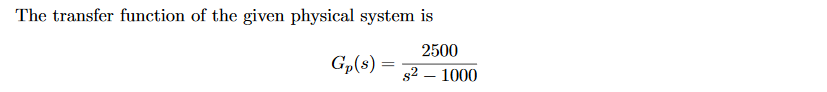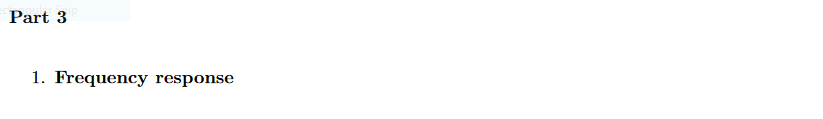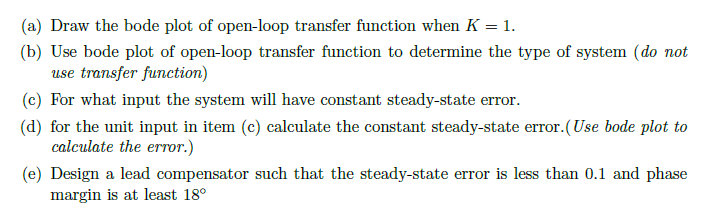The transfer function of the given physical system is 2500 Gp(s)-T-1000
Part 3 1. Frequency response
(a) Draw the bode plot of open-loop transfer function when K (b) Use bode plot of open-loop transfer function to determine the type of system (do not use transfer function) (c) For what input the system will have constant steady-state error (d) for the unit input in item (c) calculate the constant steady-state error.(Use bode plot to calculate the error.) (e) Design a lead compensator such that the steady-state error is less than 0.1 and phase margin is at least 18°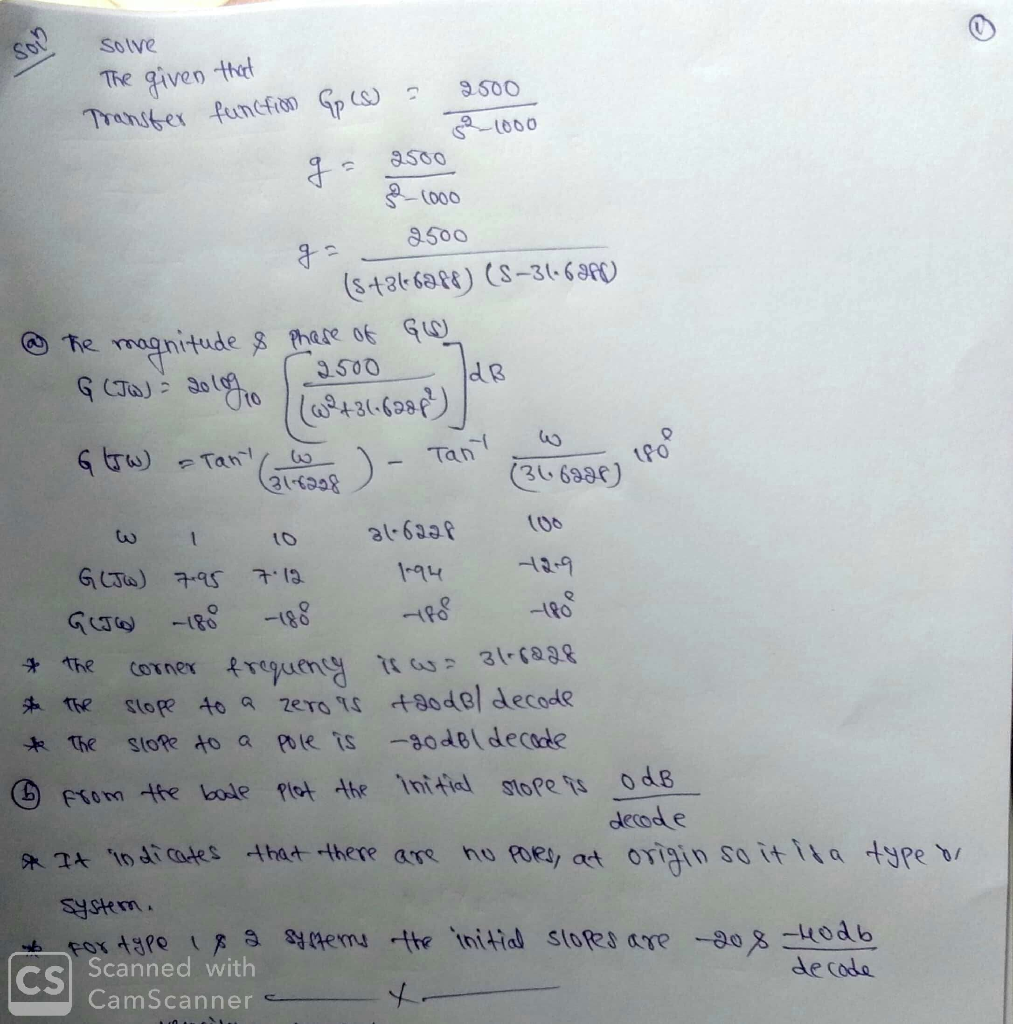#### Earn Coins

Coins can be redeemed for fabulous gifts.

Similar Homework Help Questions
• ### Please show all work and write neatly so I can understand how to do this on my own. Thank you. The transfer function of the given physical system is 2500 Gp(s)- P21000 The physical system is controll...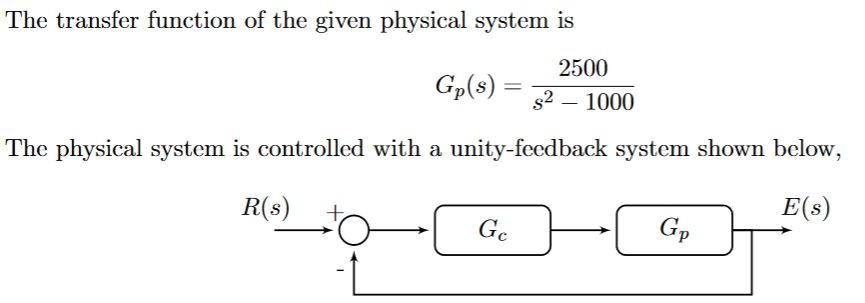Please show all work and write neatly so I can understand how to do this on my own. Thank you. The transfer function of the given physical system is 2500 Gp(s)- P21000 The physical system is controlled with a unity-feedback system shown below, E(s) R(s) + (e) For what input the system will have constant steady-state error. (d) for the unit input in item (c) calculate the constant steady-state error.(Use bode plot to calculate the error.) The transfer function of...

• ### The transfer function of the given physical system is Gp(s)-1000 The physical system is controlle...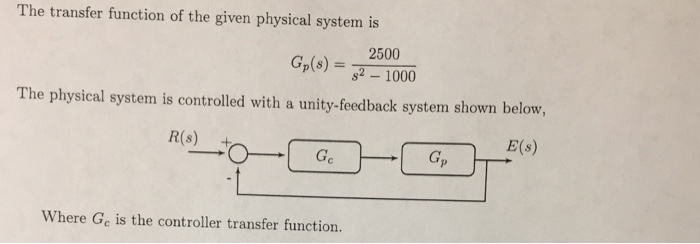The transfer function of the given physical system is Gp(s)-1000 The physical system is controlled with a unity-feedback system shown below, R(s) + Where Ge is the controller transfer function 3. Lead/Lag Compensator (a) Design a compensator such that the settling time of the compensated system T < 0.02 sec (Use 5% definition), and maximum overshoot of the compensated system is Mp 20%. Clearly explain all your steps. (b) Build a simulink model and use the compensator you designed above....

• ### Consider the system shown as below. Draw a Bode diagram of the open-loop transfer function G(s).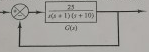1 Consider the system shown as below. Draw a Bode diagram of the open-loop transfer function G(s). Determine the phase margin, gain-crossover frequency, gain margin and phase-crossover frequency, (Sketch the bode diagram by hand) 2 Consider the system shown as below. Use MATLAB to draw a bode diagram of the open-loop transfer function G(s). Show the gain-crossover frequency and phase-crossover frequency in the Bode diagram and determine the phase margin and gain margin. 3. Consider the system shown as below. Design a...

• ### Please show all work and write neatly so I can understand how to do this on my own. Thank you. The transfer function of the given physical system is 2500 Gp(s)- P21000 The physical system is controll...Please show all work and write neatly so I can understand how to do this on my own. Thank you. The transfer function of the given physical system is 2500 Gp(s)- P21000 The physical system is controlled with a unity-feedback system shown below, E(s) R(s) + (a) Draw the bode plot of open-loop transfer function when K-1. (b) Use bode plot of open-loop transfer function to determine the type of system (do not use transfer function) The transfer function of...

• ### P4) Consider a system with open loop transfer function of G(s) ? a) Sketch the Bode...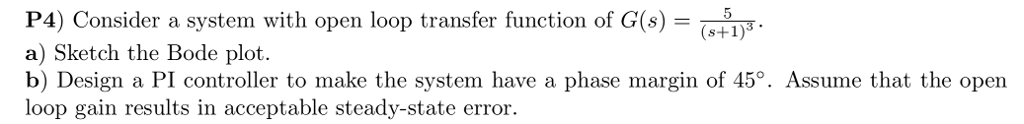P4) Consider a system with open loop transfer function of G(s) ? a) Sketch the Bode plot. b) Design a PI controller to make the system have a phase margin of 45°. Assume that the open loop s+1)3 gain results in acceptable steady-state error

• ### Bode Diagram 10 10 Frequency (rad/s) Bode Diagram 100F 140 10 10 Frequency (rad/s) Figure Q4.2 4. The de servo system s...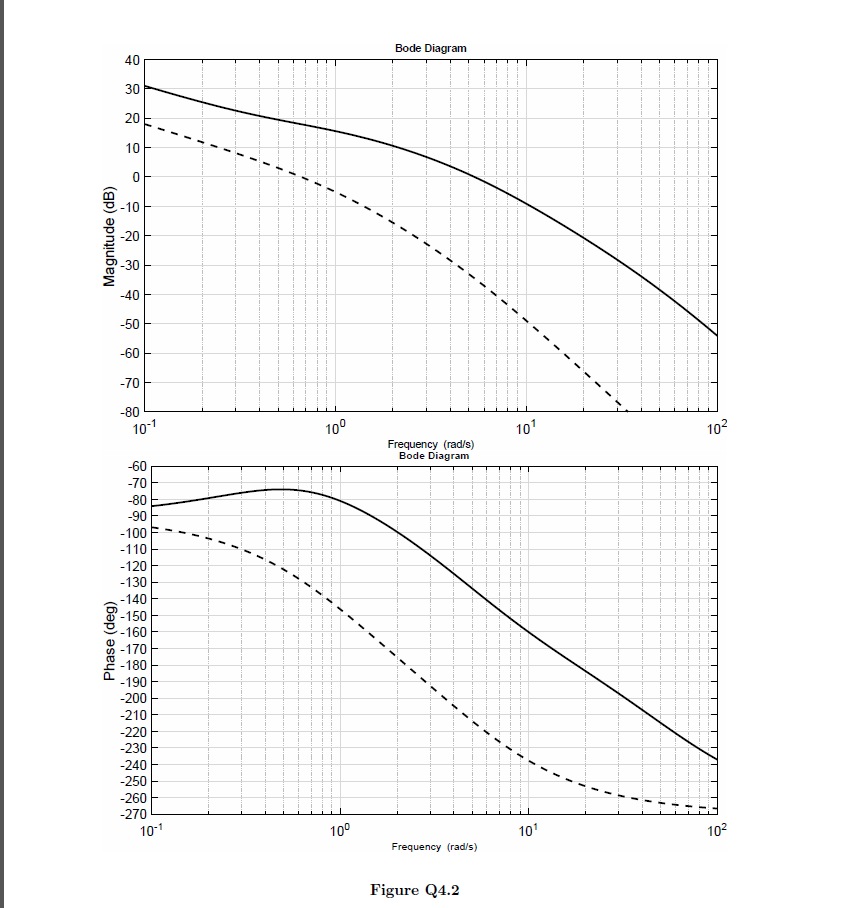Bode Diagram 10 10 Frequency (rad/s) Bode Diagram 100F 140 10 10 Frequency (rad/s) Figure Q4.2 4. The de servo system shown in Figure Q4.1 is required to have a transient step response speci fication with a peak time of 0.58 seconds or better, and a +2% setting time of 1.7 seconds or better 01(s) K (s)G(s) s(s 1 (s 5) Figure Q4.1 The Bode diagram of the open-loop system is shown in Figure Q4.2 on page 8. This Bode...

• ### design a lead compensator For the system with the following open loop transfer function, G(S) (05s+1 Design a lead c...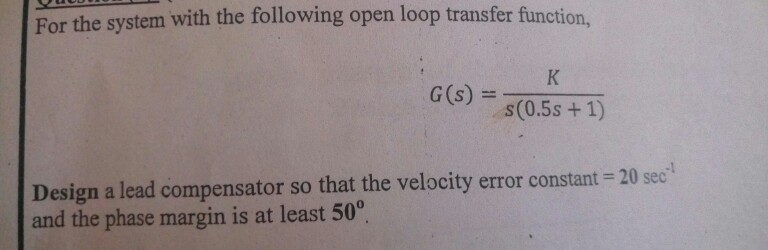design a lead compensator For the system with the following open loop transfer function, G(S) (05s+1 Design a lead compensator so that the velocity error constant 20 sec and the phase margin is at least 50° For the system with the following open loop transfer function, G(S) (05s+1 Design a lead compensator so that the velocity error constant 20 sec and the phase margin is at least 50°

• ### Consider a system modelled by means of the following transfer function 10 G(s) s(s +1)(s +10)...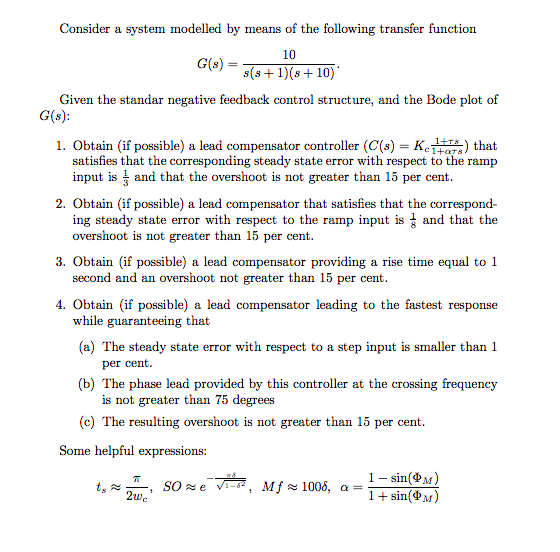Consider a system modelled by means of the following transfer function 10 G(s) s(s +1)(s +10) Given the standar negative feedback control structure, and the Bode plot of G(s): 1. Obtain (if possible) a lead compensator controller (C(s) Kc1+ts) that satisfies that the corresponding steady state error with respect to the ramp input is and that the overshoot is not greater than 15 per cent 2. Obtain (if possible) a lead compensator that satisfies that the correspond- ing steady state...

• ### Q.4 A position control system is shown in Figure Q4. Assume that K(s) = K, the plant 50 s(0.2s +1) transfer function is given by G(s) s02s y(t) r(t) Figure Q4: Feedback control system. (a) Design a l...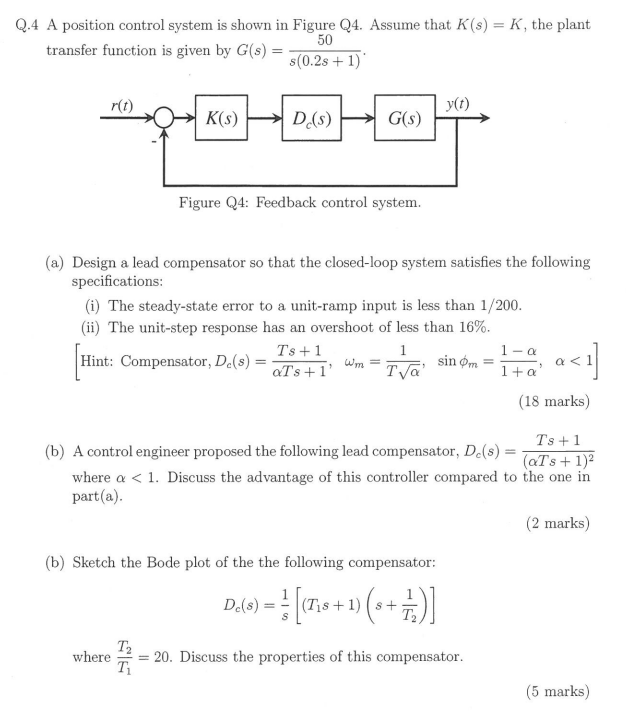Q.4 A position control system is shown in Figure Q4. Assume that K(s) = K, the plant 50 s(0.2s +1) transfer function is given by G(s) s02s y(t) r(t) Figure Q4: Feedback control system. (a) Design a lead compensator so that the closed-loop system satisfies the following specifications (i) The steady-state error to a unit-ramp input is less than 1/200 (ii) The unit-step response has an overshoot of less than 16% Ts +1 Hint: Compensator, Dc(s)=aTs+ 1, wm-T (18 marks)...

• ### urgent! II Lead-Lag Controller Design A plant has the open-loop transfer function with unity feedback: 20(s +1) G, (s) s(10s +D(0.1258 +D(0.05s +1)(0.02s +1) Design a phase lag-lead compensator th...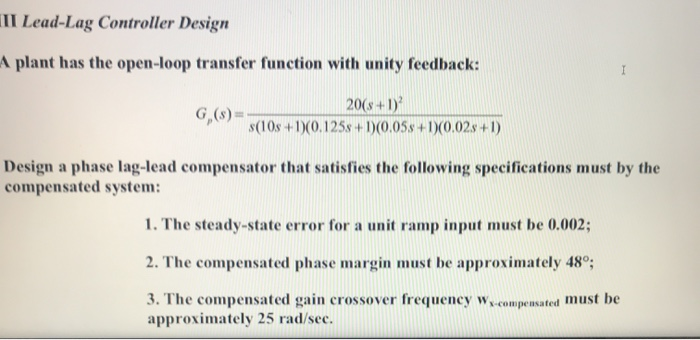urgent! II Lead-Lag Controller Design A plant has the open-loop transfer function with unity feedback: 20(s +1) G, (s) s(10s +D(0.1258 +D(0.05s +1)(0.02s +1) Design a phase lag-lead compensator that satisfies the following specifications must by the compensated system 1. The steady-state error for a unit ramp input must be 0.002; 2. The compensated phase margin must be approximately 48; must be approximately 25 rad/sec. II Lead-Lag Controller Design A plant has the open-loop transfer function with unity feedback: 20(s...